# ML Aggarwal Class 7 Solutions for ICSE Maths Chapter 11 Triangles and its Properties Check Your Progress

## ML Aggarwal Class 7 Solutions for ICSE Maths Chapter 11 Triangles and its Properties Check Your Progress

Question 1.
Find the value of x in each of the following diagrams: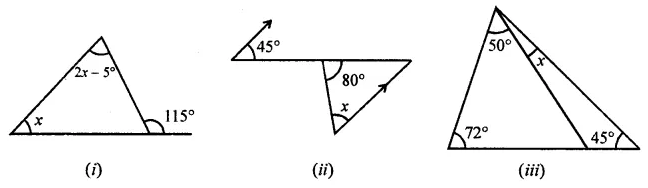Solution: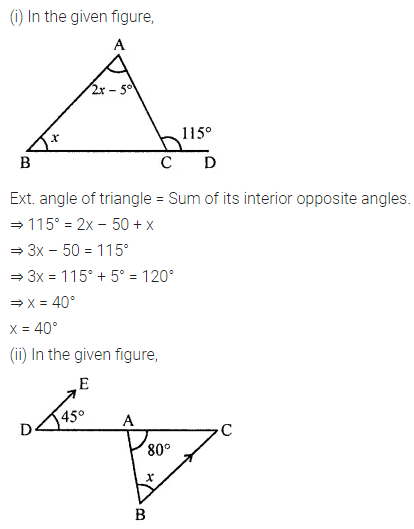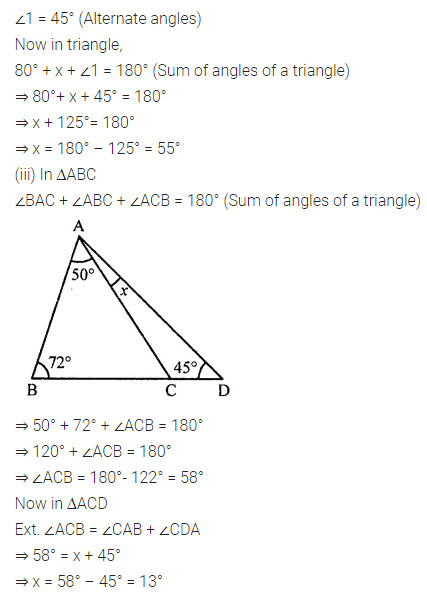Question 2.
In the given figure, ∠B = 70° and ∠A = 50°. If the bisector of ∠C meets AB in D, then find ∠ADC.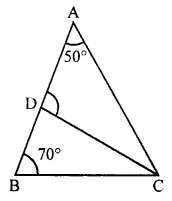Solution: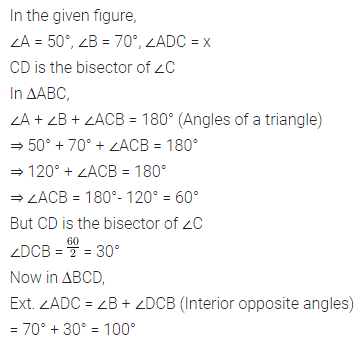Question 3.
Find the values of x and y in each of the following figures:Solution: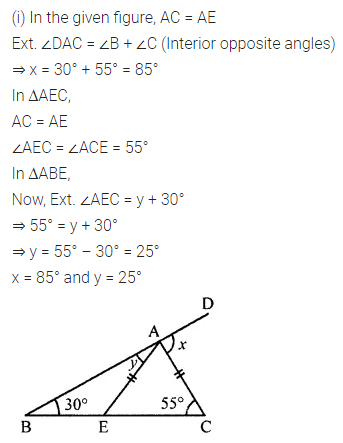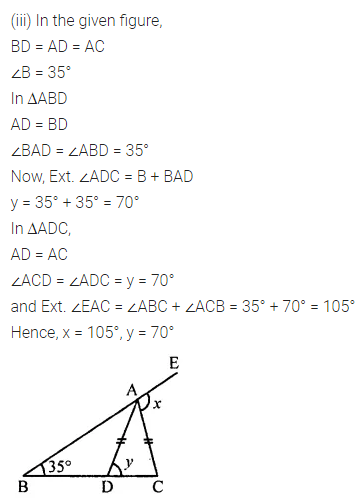Question 4.
If the two acute angles of a right-angled triangle are in the ratio 7 : 8, find these angles.
Solution: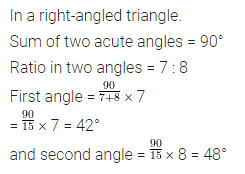Question 5.
If the angles of a triangle are (3x)°, (2x – 7)° and (4x – 11)°, then find the value of x.
Solution: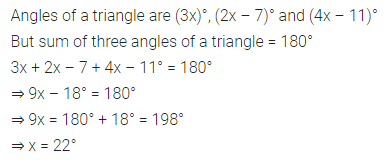Question 6.
In an isosceles triangle, the vertical angle is 15° greater than each of its base angles. Find all the angles of the triangle.
Solution: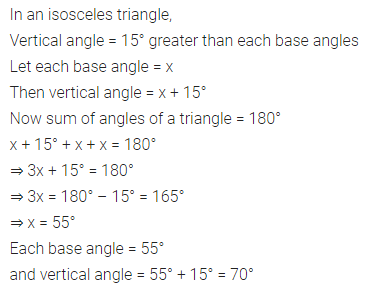Question 7.
Can a triangle have three sides whose lengths are
(i) 4.5 cm, 3.8 cm, 7.2 cm?
(ii) 3.2 cm, 5.3 cm, 9.4 cm?
Solution: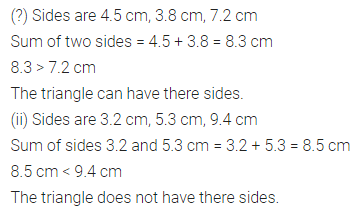Question 8.
If the lengths of two sides of a triangle are 5 cm and 12 cm, then what can be the length of the third side?
Solution:Question 9.
In the given figure, all measurements are in centimeters. If AD is perpendicular to BC, find the length of AB.Solution:Question 10.
In the given figure, AB and CD are two vertical poles of height 19 m and 11 m respectively. If the shortest distance between their tops is 17 m, find how far apart they are?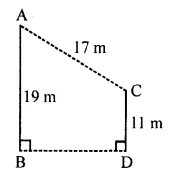Solution: